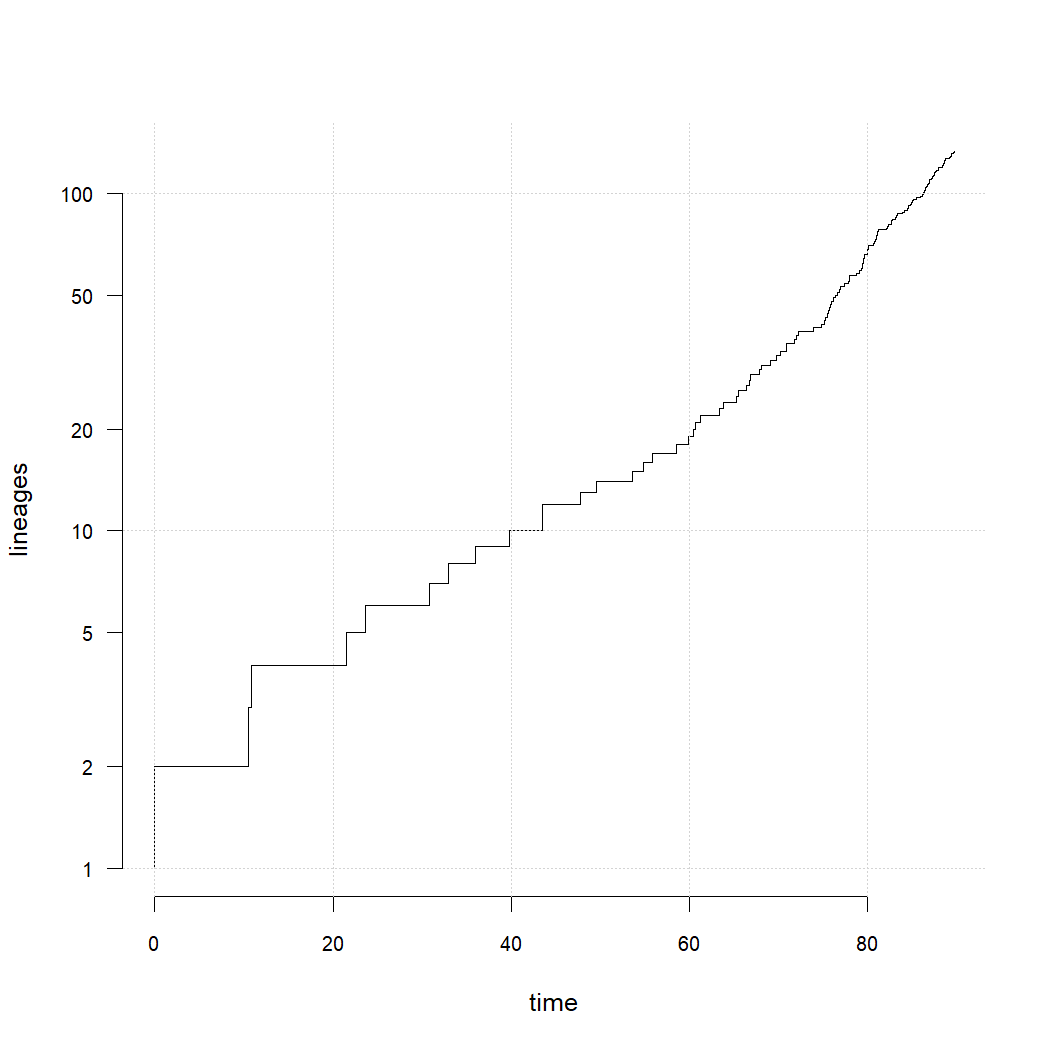## Wednesday, August 24, 2022

### An `anova` method for the `"fit.bd"` object class (and how to write your own)

Over the past couple of days I've either shown or used new S3 generic `anova` methods for different phytools object classes.

These are relatively straightforward to write, and since they can make model comparison in phytools (sometimes) much easier, I thought I'd illustrate an example function.

Here is an `anova` method for the object class `"fit.bd"`. (`"fit.bd"` objects are created by the phytools functions `fit.bd`, for fitting a birth-death model with incomplete taxon sampling to a reconstructed phylogeny; and `fit.yule`.)

I'll add this to phytools shortly.

``````anova.fit.bd<-function(object,...){
fits<-list(...)
nm<-c(
deparse(substitute(object)),
if(length(fits)>0)
sapply(substitute(list(...))[-1],deparse)
)
fits<-c(list(object),fits)
logL<-sapply(fits,logLik)
df<-sapply(fits,function(x) attr(logLik(x),"df"))
AIC<-sapply(fits,AIC)
weight<-unclass(aic.w(AIC))
result<-data.frame(logL,df,AIC,weight)
rownames(result)<-nm
colnames(result)<-c("log(L)","d.f.","AIC","weight")
print(result)
invisible(result)
}
``````

Now let's use it with a simulated case in which the true death (extinction) rate is exactly half the birth (speciation) rate.

``````tree<-pbtree(b=0.1,d=0.05,t=100)
tree<-drop.tip(tree,getExtinct(tree))
plot(ltt(tree,plot=FALSE),bty="n",log.lineages=FALSE,
log="y",cex.axis=0.8,las=1)
grid()
``````Fit our models.

``````birth_death<-fit.bd(tree)
``````
``````## Warning in nlminb(c(init.b, init.d), lik.bd, t = T, rho = rho, lower = rep(0, : NA/NaN function evaluation
``````
``````birth_death
``````
``````##
## Fitted birth-death model:
##
## ML(b/lambda) = 0.08452
## ML(d/mu) = 0.04266
## log(L) = 22.6402
##
## Assumed sampling fraction (rho) = 1
##
## R thinks it has converged.
``````
``````yule<-fit.yule(tree)
yule
``````
``````##
## Fitted Yule model:
##
## ML(b/lambda) = 0.06173
## log(L) = 20.0631
##
## Assumed sampling fraction (rho) = 1
##
## R thinks it has converged.
``````

Compare using new `anova` method.

``````anova(yule,birth_death)
``````
``````##               log(L) d.f.       AIC    weight
## yule        20.06310    1 -38.12619 0.1712015
## birth_death 22.64023    2 -41.28046 0.8287985
``````